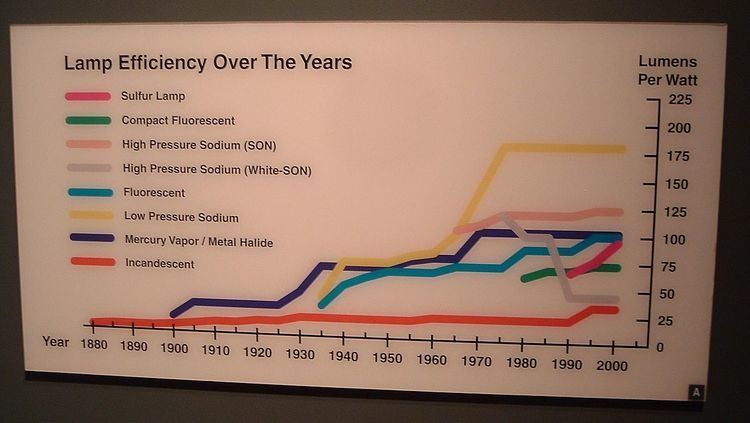# Electrical efficiency

Updated on
Covid-19The efficiency of an entity (a device, component, or system) in electronics and electrical engineering is defined as useful power output divided by the total electrical power consumed (a fractional expression), typically denoted by the Greek small letter eta (η – ήτα).

## Contents

E f f i c i e n c y = U s e f u l   p o w e r   o u t p u t T o t a l   p o w e r   i n p u t

If energy output and input are expressed in the same units, efficiency is a dimensionless number. Where it is not customary or convenient to represent input and output energy in the same units, efficiency-like quantities have units associated with them. For example, the heat rate of a fossil-fuel power plant may be expressed in BTU per kilowatt-hour. Luminous efficacy of a light source expresses the amount of visible light for a certain amount of power transfer and has the units of lumens per watt.

## Efficiency of typical electrical devices

Efficiency should not be confused with effectiveness: a system that wastes most of its input power but produces exactly what it is meant to is effective but not efficient. The term "efficiency" makes sense only in reference to the wanted effect. A light bulb, for example, might have 2% efficiency at emitting light yet still be 98% efficient at heating a room (In practice it is nearly 100% efficient at heating a room because the light energy will also be converted to heat eventually, apart from the small fraction that leaves through the windows). An electronic amplifier that delivers 10 watts of power to its load (e.g., a loudspeaker), while drawing 20 watts of power from a power source is 50% efficient. (10/20 × 100 = 50%)

• Electric kettle: more than 90% (comparatively little heat energy is lost during the 2 to 3 minutes a kettle takes to boil water).
• A premium efficiency electric motor: more than 90% (see Main Article: Premium efficiency).
• A large power transformer used in the electrical grid may have efficiency of more than 99%. Early 19th century transformers were much less efficient, wasting up to a third of the energy passing through them.
• ## Efficiency of devices at point of maximum power transfer ...

As a result of the maximum power theorem, devices transfer maximum power to a load when running at 50% electrical efficiency. This occurs when the load resistance (of the device in question) is equal to the internal Thevenin equivalent resistance of the power source. This is valid only for non-reactive source and load impedances.

## Efficiency of light bulbs

• Incandescent light bulb: about 2%.
• Compact fluorescent lamp: about 7–9%.
• White light-emitting diode (LED) about 4–18%.
• ## Discussion

High efficiency is particularly relevant in systems that can operate from batteries. Inefficiency may require weighing the cost either of the wasted energy, or of the required power supply, against the cost of attaining greater efficiency. Efficiency can usually be improved by choosing different components or by redesigning the system. Inefficiency probably produces extra heat within the system, which must be removed if it is to remain within its operating temperature range. In a climate-controlled environment, like a home or office, heat generated by appliances may reduce heating costs or increase air conditioning costs.

## References

Similar Topics
Benjamin Balleret
Andrés Pelussi
Scott Maginness
Topics